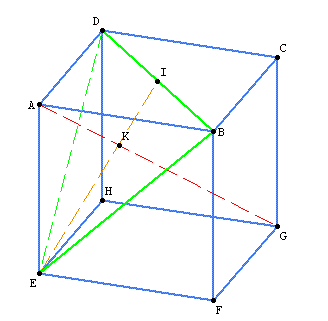2023-08-19 07:39:06 +0200 received badge ● Famous Question (source) 2017-10-07 11:25:10 +0200 received badge ● Notable Question (source) 2016-07-27 06:19:11 +0200 received badge ● Popular Question (source) 2013-12-15 15:31:47 +0200 received badge ● Student (source) 2013-12-15 15:26:45 +0200 commented answer Compute the volume of a cube region Thank you a lot... Sage is powerful yet so simple :) 2013-12-15 15:26:05 +0200 marked best answer Compute the volume of a cube region You can construct the 3-dimensional cube as follows: sage: P = polytopes.n_cube(3) ; P A 3-dimensional polyhedron in ZZ^3 defined as the convex hull of 8 vertices sage: P.vertices() (A vertex at (-1, -1, -1), A vertex at (-1, -1, 1), A vertex at (-1, 1, -1), A vertex at (-1, 1, 1), A vertex at (1, -1, -1), A vertex at (1, -1, 1), A vertex at (1, 1, -1), A vertex at (1, 1, 1))  Then construct the polyhedron with the last vertex removed: sage: Q = Polyhedron(P.vertices()[:-1]) ; Q A 3-dimensional polyhedron in ZZ^3 defined as the convex hull of 7 vertices sage: Q.vertices() (A vertex at (-1, -1, -1), A vertex at (-1, -1, 1), A vertex at (-1, 1, -1), A vertex at (-1, 1, 1), A vertex at (1, -1, -1), A vertex at (1, -1, 1), A vertex at (1, 1, -1)) Then compute its volume: sage: Q.volume() 20/3  In our case, the length of the edge is 2. If you want to replace it by a, it suffice to multipliy the result by by (a/2)^3: sage: a = var('a') sage: Q.volume()* (a/2)^3 5/6*a^3  If you want to have a plot of the polyhedron Q, just do: sage: Q.plot()  2013-12-15 15:26:05 +0200 received badge ● Scholar (source) 2013-12-15 15:25:58 +0200 received badge ● Supporter (source) 2013-12-15 14:16:57 +0200 received badge ● Editor (source) 2013-12-15 14:15:52 +0200 asked a question Compute the volume of a cube region Hi, I would like to compute the volume $V$ of the lower polyhedron (that does not contain vertex A) let's call it $poly_G$. (let's call $poly_A$ the upper polyhedron that does not contain G). Size is $a=AB=BF=...$We can compute $V_{poly_A}$ by introducing K and I points and using Pythagore's theorem and median's properties . I can do that by hand using $V_{poly_G}=V_{cube}-V_{poly_A}$ I want to compute the volume with sage, I have tried this code using volume integrations but I am not sure about the result at all... I look at first for the normal vector of plane DBE (cross product) which gives the equation plane $x-y+z$. Then I compute using triple integrations. x=var('x') y=var('y') z=var('z') a=var('a') u=vector([a,a,0]) v=vector([0,a,a]) print u.cross_product(v) intz = integrate(1,z,0,a-y-x) inty = integrate(intz,y,0,a-x) intx = integrate(inty,x,0,a) print intx  I would like to know if there are elegant ways of computing this kind of problem in sage ? And in addition, if this require few lines, how can I display the above figure ? Sorry if this looks simple, I am new to Sage. Thanks,